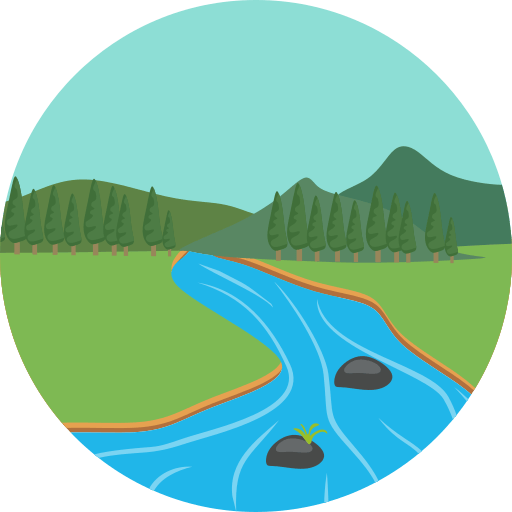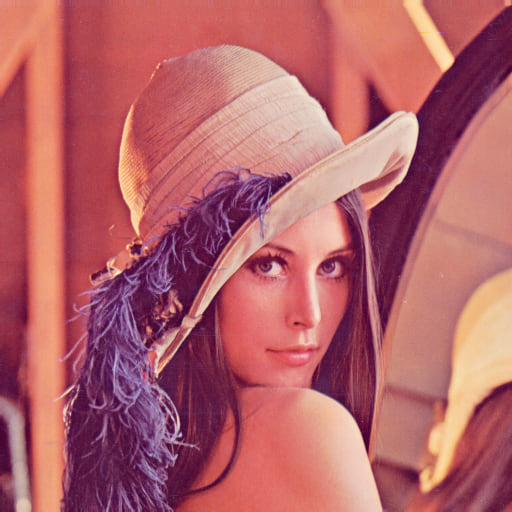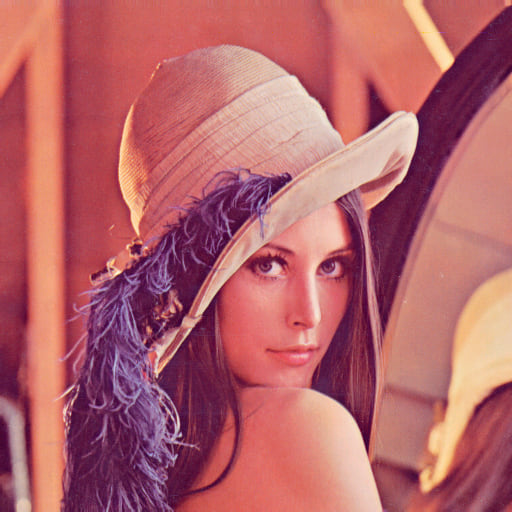## greenfield

A library for greenfield images

### 3 releases

 0.1.4 Jan 28, 2023 Jan 28, 2023 Jan 21, 2023

Used in greenriver

115KB
972 lines# Greenfield

A Rust implementation of the greenfield image format

## The greenfield image format

The greenfield image format is a simple 2D array of colors, prefixed with the width and height of the image, and a quantization information. All of the field are stored in big endian format.

• The first 64 bits(a full u64) are the greenfield magic value, used to identify the file as a greenfield image (`b"grnfld42"`).
• The next 16 bits (a full u64) bits are the width of the image.
• The next 16 bits (a full u64) bits are the height of the image.
• The next 12 bits are the quantization information tuple (see [`quantization`]). A qunatization tuple is in the form: `(bits_r, bits_g, bits_b)`, where each value is the number of bits used to store the respective color component.
• The remaining bits are the image color data, in row-major order. Each color has (bits_r + bits_g + bits_b) bits. So, for example, if the quantization tuple is `(5, 6, 5)`, then each color is 16 bits. To get all the colors, you must read (width height) (bits_r + bits_g + bits_b) bits.

## Format on Disk

``````╔════════════════════════════╤══════════════════════════════════════════════════════════╗
║            Bits            │                      Description                         ║
╠════════════════════════════╪══════════════════════════════════════════════════════════╣
║             64             │      b"grnfld42": Magic value (0x47524E464C443432)       ║
╟────────────────────────────┼──────────────────────────────────────────────────────────╢
║             16             │                   u32: Image width                       ║
╟────────────────────────────┼──────────────────────────────────────────────────────────╢
║             16             │                   u32: Image height                      ║
╟────────────────────────────┼──────────────────────────────────────────────────────────╢
║             12             │      (bits_r, bits_g, bits_b): Quantization tuple        ║
╟────────────────────────────┼──────────────────────────────────────────────────────────╢
║      width * height *      │       [RGB]: (bits_r + bits_g + bits_r) per pixel        ║
║ (bits_r + bits_g + bits_b) │                       row-major                          ║
╚════════════════════════════╧══════════════════════════════════════════════════════════╝
``````

## Color Quantization

Rgb quantization is the process of reducing the color space size of an image with the objective of reducing the size of the image in disk. This is done by grouping similar colors in the space and referring to the group instead of a particular color.

Greenfield images uses a quantization technique know as Uniform Quantization. In uniform quantization, we divide each component of the color space in equal intervals, indexing each interval with a number, and then we assign a color to each interval (usually the mean of this interval), in the end, we just store on disk this index, instead of the color. With that, we can reduce the number of bits needed to store each component of a color. Once an image has been loaded from disk, we can find the respective color for each index (the mean of an interval represented by that index) and reconstruct the image.

For example, if we have a 24-bit RGB image, we can reduce the number of bits needed to store each component to 5 bits, reducing the number of bits needed to store each pixel from 24 to 15. Reducing each component to 5 bits, we now have 2^5 = 32 possible values for each component. Each distinct value is the mean of the interval in the RGB color space.

## Installation

This library is available on crates.io/crates/greenfield. So, just install it with cargo:

``````cargo add greenfield
``````

## Usage

### Basic Operations

``````use std::error::Error;

use greenfield::prelude::*;

fn main() -> Result<(), Box<dyn Error>> {
// Creation of images
let image = Image::new(
1,
1,
UniformQuantization::new(5, 6, 5)?,
vec![Rgb::new(255, 255, 255)],
)?;
println!("Image: {}", image);

// Fields
let (width, height) = image.dimensions();
let quantization = image.quantization();

println!("Dimensions: {}x{}", width, height);
println!("Quantization: {}", quantization);

// Iterators over image data
let colors = image.colors().collect::<Vec<&Rgb>>(); // Vec<Item = Rgb>
let pixels = image.pixels(); // Iter<Item = Pixel>
let bytes = image.bytes(); // Iter<Item = u8>

for color in colors {
println!("Color: {}", color);
}

for pixel in pixels {
println!("Pixel: {}", pixel);
}

for byte in bytes {
println!("Byte: {}", byte);
}

Ok(())
}
``````

### Example quantization

Lenna image quantized to 5 6 5 bits.### Serialization/Deserialization

This crate use the deku for serialization and deserialization operations.

I've provided some utility methods on top of that for conversions between greenfield images and bytes:

``````use std::{error::Error, path::PathBuf};

use greenfield::prelude::*;

fn main() -> Result<(), Box<dyn Error>> {
let image = Image::new(
1,
1,
UniformQuantization::new(8, 8, 8)?,
vec![Rgb::new(255, 255, 255)],
)?;

// to/from bytes
let serialized = image.clone().serialize()?;
let deserialized = Image::deserialize(&serialized)?;
println!("{}", deserialized);

// to/from file
image.to_file(&PathBuf::from("image.gfd"))?;
let img = Image::from_file(&PathBuf::from("image.gfd"))?;
println!("{}", img);

Ok(())
}
``````

### Conversion between common formats

For conversion between gfd files and other formats (e.g. png and bmp) the crate image is used.

I have provided the functions `greenfield::io::{load_image, and save_image}` for simplicity sake. Note that the formats used for saving or loading are inferred from filename extensions (e.g. .png saves as png and.gfd as greenfield).

``````use std::{error::Error, path::PathBuf};

use greenfield::prelude::*;

fn main() -> Result<(), Box<dyn Error>> {
// Load a PNG an save as GFD
&PathBuf::from("Lenna.png"),
UniformQuantization::new(8, 8, 8)?,
)?;
save_image(&lenna, &PathBuf::from("Lenna.gfd"))?;

// Load a GFD and save as PNG